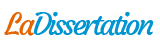# Arithmétique de base (document en anglais)

Analyse sectorielle : Arithmétique de base (document en anglais). Recherche parmi 237 000+ dissertations

Par   •  19 Décembre 2014  •  Analyse sectorielle  •  206 Mots (1 Pages)  •  285 Vues

2 Basic Arithmetic

2.3 Indices

Indices or powers starts of as repeated multiplication. In this chapter we will look at how indices are written and state the rules which are used for manipulating them.

2.3.1 Index Notation

If is a real number and is a natural number then

.

is called the index, power or exponent on and is called the base.

Example:

The number is written, for short as and we read it as “6 raised to the power 3” or “6 cubed”.

When dealing with numbers your calculator is able to evaluate expressions involving powers. Using the power button on your calculator you should obtain .

Example:

Identify the base and the index:

In the expression , 7 is the base and 12 is the index.

In the expression , 3 is the base and 5 is the index.

In the expression , 2 is the base and is the index.

2.3.2 Rules for Indices

add indices when multiplying numbers or

variables with the same base

subtract indices when dividing numbers or variables with the same base

multiply indices together when raising a number or variables to a power

Examples:

Use the laws of indices:

...